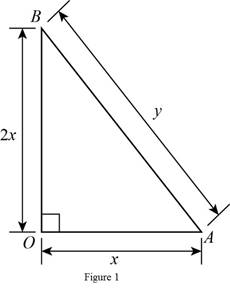# The function that models the perimeter of right triangle in terms of its shorter length whose one leg is twice as long as other leg.### Precalculus: Mathematics for Calcu...

6th Edition
Stewart + 5 others
Publisher: Cengage Learning
ISBN: 9780840068071### Precalculus: Mathematics for Calcu...

6th Edition
Stewart + 5 others
Publisher: Cengage Learning
ISBN: 9780840068071

#### Solutions

Chapter 2, Problem 16P
To determine

## To find: The function that models the perimeter of right triangle in terms of its shorter length whose one leg is twice as long as other leg.

Expert Solution

The function that model the perimeter is P(x)=x(3+5).

### Explanation of Solution

Draw the diagram of right triangle as shown in the figure below.Let OA be x, AB be y and as per given condition OB is 2x.

Apply Pythagorean Theorem in triangle AOB

(AB)2=(OA)2+(OB)2

Substitute y for AB, x for OA and 2x for OB in above equation.

(y)2=(x)2+(2x)2y2=x2+4x2y2=5x2

Take square root of above equation.

y=5x

Perimeter of a triangle is sum of all the sides.

P=x+2x+y=3x+y

Substitute 5x for y in above equation.

P=3x+5x=x(3+5)

Thus, the function that models the perimeter of the triangle is P(x)=x(3+5).

### Have a homework question?

Subscribe to bartleby learn! Ask subject matter experts 30 homework questions each month. Plus, you’ll have access to millions of step-by-step textbook answers!